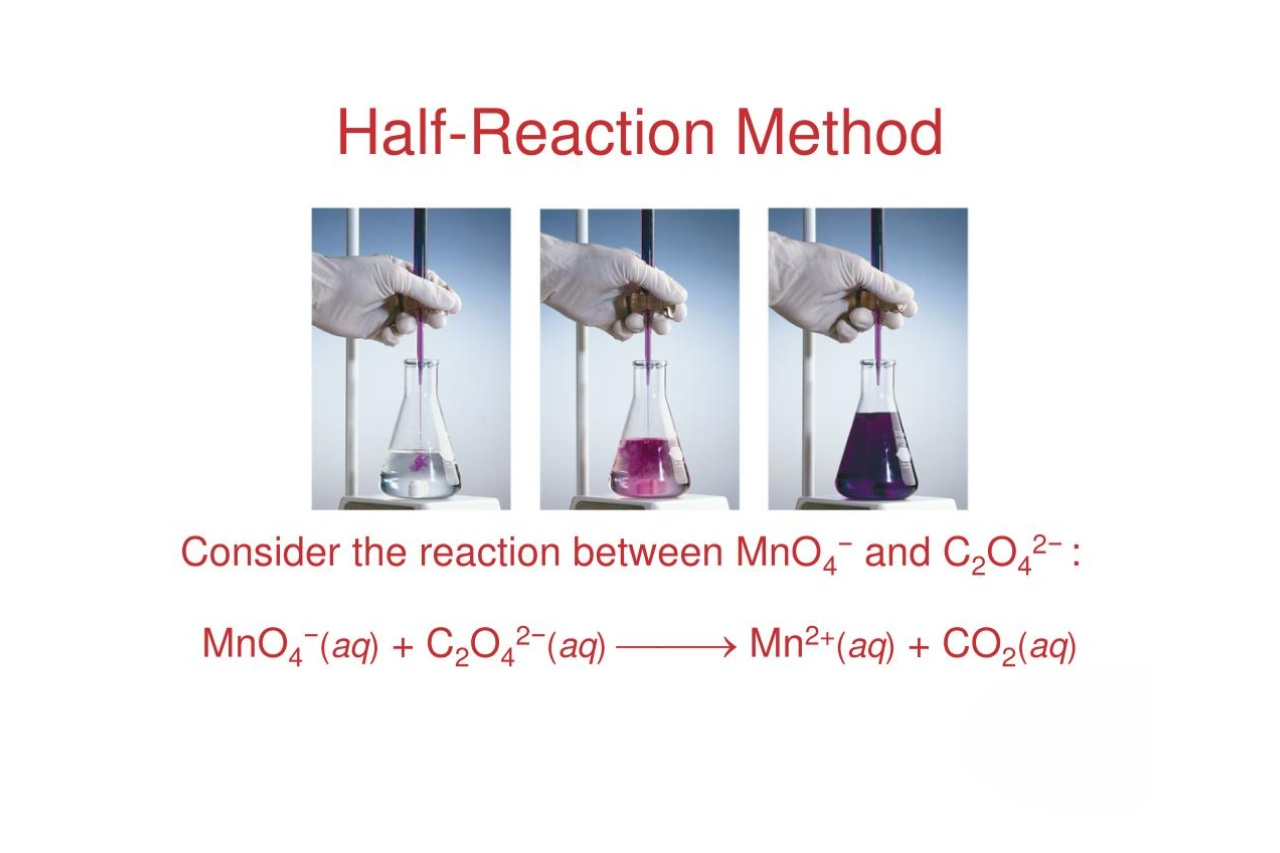## Latest Facts

### 17 Intriguing Facts About 3D ModelingDorris Lafontaine

Published: 11 Sep 2023Source: Slideserve.com

Chemistry is a fascinating and intricate field of science that explores the behavior and properties of matter. Within this vast subject lies the concept of redox reactions, which involve the transfer of electrons between different chemical species. One important aspect of redox reactions is the notion of half-reactions. These half-reactions help break down the overall redox process into smaller steps, shedding light on the individual electron transfers and oxidation-reduction behavior of each species involved. In this article, we will delve into the intriguing world of half-reactions and uncover 13 enigmatic facts that will deepen your understanding of this fundamental concept. So, join us on this journey as we unravel the mysteries behind half-reactions and explore their significance in the world of chemistry.

## Half-Reaction is a fundamental concept in chemistry.

Half-reaction, also known as half-equation, is a crucial concept in understanding redox reactions. It represents the two separate processes – oxidation and reduction – that occur simultaneously in a chemical reaction.

## Half-reaction breaks down a complex reaction into simpler steps.

By separating a redox reaction into its constituent oxidation and reduction reactions, half-reaction allows us to analyze and understand the individual electron transfer processes involved.

## Half-reaction involves the transfer of electrons.

During oxidation, electrons are lost, and during reduction, electrons are gained. Half-reaction accurately represents these electron transfers, making it easier to balance equations and calculate reaction stoichiometry.

## Half-reaction allows the determination of standard electrode potentials.

By measuring the potential difference between two half-cells, the standard electrode potential of a half-reaction can be determined. This information is valuable in predicting the feasibility and spontaneity of redox reactions.

## Half-reaction can occur separately in an electrolytic cell.

In an electrolytic cell, the oxidation and reduction half-reactions happen independently at the anode and cathode, respectively. This process is used in various electrochemical applications, including metal plating and electrolysis.

## Half-reaction is essential in balancing redox equations.

By balancing the separate oxidation and reduction half-reactions, the overall redox equation can be properly balanced to satisfy the law of conservation of mass and charge.

## Half-reaction is a mathematical representation of electron transfer.

Each half-reaction is written as a balanced chemical equation, showing the species undergoing oxidation or reduction, the transfer of electrons, and the resulting products.

## Half-reaction involves species called oxidizing agents and reducing agents.

In a redox reaction, the species that gets reduced is the oxidizing agent, while the species that gets oxidized is the reducing agent. Half-reaction helps identify and understand the role of these species in electron transfer.

## Half-reaction is used to analyze and predict redox reactions.

By calculating the standard electrode potentials of different half-reactions and comparing them, it is possible to determine which reactions are spontaneous and which are non-spontaneous.

## Half-reaction is crucial in understanding corrosion and rusting.

Corrosion and rusting involve oxidation reactions of metals. By studying the relevant half-reactions, scientists and engineers can develop strategies to prevent or minimize the damaging effects of corrosion.

## Half-reaction allows the calculation of cell potentials and Gibbs free energy changes.

By combining the standard electrode potentials of the oxidation and reduction half-reactions, the overall cell potential and the accompanying Gibbs free energy change for a redox reaction can be determined.

## Half-reaction is extensively used in the field of electrochemistry.

Electrochemistry explores the relationship between electricity and chemical reactions. Half-reaction provides a framework for understanding and quantifying these electrochemical processes.

## Half-reaction plays a crucial role in understanding biological redox reactions.

Biological systems heavily rely on redox reactions for various processes, such as energy production in cellular respiration. Understanding the underlying half-reactions is essential for studying and comprehending these bioenergetic pathways.

## Conclusion

In conclusion, half-reactions are a fascinating aspect of chemistry that play a crucial role in understanding redox reactions. They allow us to break down complex reactions into smaller, more manageable parts, making it easier to analyze and balance equations. By separating the oxidation and reduction processes, half-reactions help us identify the transfer of electrons and determine the overall electron flow in a chemical reaction.Understanding half-reactions is essential for predicting the spontaneity and feasibility of reactions, as well as for calculating cell potentials in electrochemical cells. It also provides valuable insights into the behavior of elements and compounds, allowing us to explore the world of chemical reactions on a deeper level.By delving into the enigmatic world of half-reactions, we can unravel the mysteries of redox chemistry and gain a greater understanding of the fundamental principles that govern chemical reactions.

## FAQs

Q: What is a half-reaction?

A: A half-reaction is a part of a redox reaction that shows either the oxidation or reduction process separately. It helps analyze and balance chemical equations involving electron transfer.

Q: How do you identify the oxidation and reduction processes in a half-reaction?

A: The species that loses electrons is undergoing oxidation, while the species that gains electrons is undergoing reduction.

Q: Why are half-reactions important?

A: Half-reactions allow us to understand the transfer of electrons in redox reactions, predict reaction spontaneity, and calculate cell potentials in electrochemical cells.

Q: How do you balance half-reactions?

A: Balancing half-reactions involves ensuring that the number of atoms and the charge are balanced on both the reactant and product sides.

Q: Can half-reactions occur independently?

A: Half-reactions do not typically occur independently but are part of a larger redox reaction. However, they can be independently balanced and used to analyze the electron transfer within the reaction.